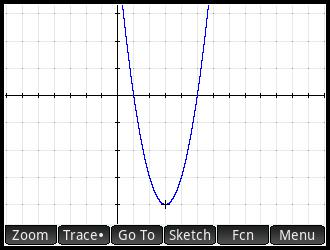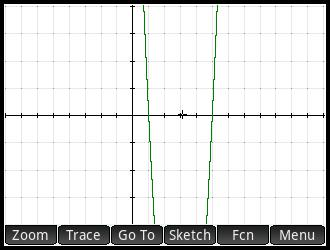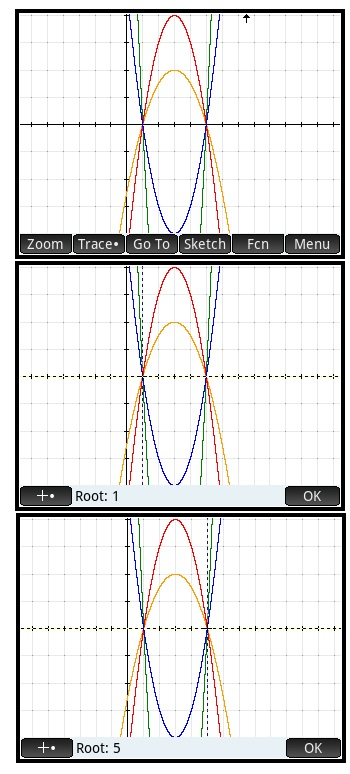## Sunday, March 11, 2018

### Forming a Quadratic Polynomial by Knowing its Roots

Forming a Quadratic Polynomial by Knowing its Roots

Introduction

We are given two roots of a quadratic equation x = A and x = B and asked to construct a quadratic polynomial.  Believe it or not, this is (almost) enough information to accomplish this task.

Since A and B are roots, that means:

0 = (x – A)*(x – B)

It’s now just a matter of some algebra:

0 = x^2 – A*x – B*x + A*B
0 = x^2 – (A+B)*x + A*B

Define this as the polynomial p(x) = x^2 – (A+B)*x + A*B

Example:  A quadratic polynomial p(x) with roots at x = 1 and x = 5.  A result is:

p(x) = x^2 – 6*x + 5x^2 - 6*x + 5.  All screen shots are generated from the HP Prime Emulator

Note that this polynomial is concave up for all x in the real numbers.  In calculus, a function is concave up when the second derivative is positive.  We can imagine bucket holding water.

Yes, observe:

0 = (x – A)*(x – B)
0 = x^2 – (A+B)*x + A*B

Now multiply both sides by -1:

0 = -x^2 + (A+B)*x – A*B

Note that 0 * -1 = 0.

Let’s name this quadratic polynomial q(x) = -x^2 + (A+B)*x – A*B.

Going back to our example, with roots x = 1 and x = 5, q(x) is defined as:
q(x) = -x^2 + 6*x – 5-x^2 + 6*x - 5

In this case this polynomial is concave down for all x.  It’s like the bucket has been turned upside down and water is spilling.

Can there be any Other Polynomials?

0 = (x – A)*(x – B)

Multiply by sides by an amplifying factor C:

C * 0 = C * (x – A) * (x – B)
0 = C * (x^2 – (A + B)*x + A*B)
0 = C * x^2 – C*(A + B)*x + A*B*C

Name this polynomial r(x) = C * x^2 – C*(A + B)*x + A*B*C

Back to the example, where roots are located at x = 1 and x = 5, let’s assume an amplifying factor of C = 3.  The result is:

r(x) = 3*x^2 – 18*x + 153*x^2 - 18*x + 15
The following is a set of four quadratic polynomials that can be formed with roots x = 1 and x = 5:Four quadratic polynomials with roots x = 1 and x = 5

 Polynomial Values Color (see above) p(x) = x^2 – 6*x + 5 A = 1, B = 5, C = 1; concave up Blue q(x) = -x^2 + 6*x – 5 A = 1, B = 5, C = -1; concave down Red r(x) = 3*x^2 – 18*x + 15 A = 1, B = 5, C = 3; concave up Green s(x) = -1/2*x^2 + 3*x – 5/2 A = 1, B = 5, C = -1/2; concave down Orange

Summary

Given the roots of the quadratic polynomial x = A and x = B, with its amplifying factor C, possible quadratic polynomials can be formed by:

f(x) = C * x^2 – C*(A + B)*x + A*B*C

If C = 1, this simplifies to:  f(x) = x^2 – (A + B)*x + A*B

If C = -1, this simplifies to:  f(x) = -x^2 + (A + B)*x - A*B

If C>0, the second derivative is positive (f’’(x) = 2*C) and the polynomial is concave up.

If C<0, the second derivative is negative (f’’(x) = -2*C) and the polynomial is concave down.

Eddie

This blog is property of Edward Shore, 2018.

#### 1 comment:

1.### Retro Review: TI-95 Procalc

Retro Review:  TI-95 Procalc  This review covers the calculator itself, not any of the optional accessories which includes a ...